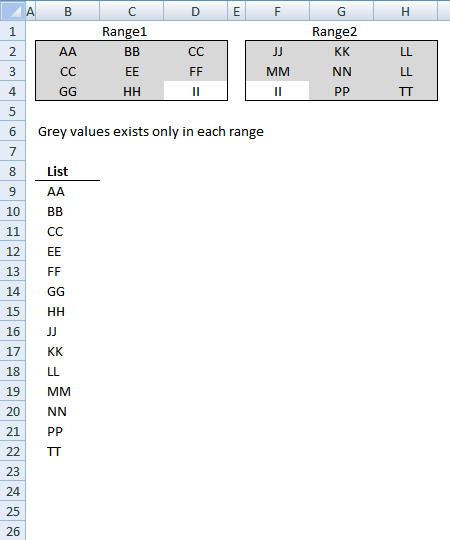Author: Oscar Cronquist Article last updated on January 07, 2019This post describes a custom function (User defined Function) that extract values existing only in one out of two cell ranges, see picture above. For example, value II is not extracted because it exists in both cell ranges Range1 and Range2.

This UDF is useful if you have lots of data and a formula is too slow.

Array formula in cell range B9:B22:

=Filter_Values(B2:D4, F2:H4)

To enter an array formula, type the formula in a cell then press and hold CTRL + SHIFT simultaneously, now press Enter once. Release all keys.

The formula bar now shows the formula with a beginning and ending curly bracket telling you that you entered the formula successfully. Don't enter the curly brackets yourself.

Note: This User defined Function is not case sensitive meaning AA and aa is considered to be the same value.

### Excel user defined function

```Function Filter_Values(rng1 As Variant, rng2 As Variant) As Variant
' This udf filter values that exists only in one out of two ranges
Dim Value As Variant
Dim temp() As Variant
Dim Test1 As New Collection
Dim Test2 As New Collection
ReDim temp(0)

rng1 = rng1.Value
rng2 = rng2.Value

On Error Resume Next
For Each Value In rng1
If Len(Value) > 0 Then Test1.Add Value, CStr(Value)
Next Value

For Each Value In rng2
If Len(Value) > 0 Then Test2.Add Value, CStr(Value)
Next Value

Err = False
For Each Value In Test2
If Len(Value) > 0 Then Test1.Add Value, CStr(Value)
If Err Then
Test1.Remove Value
End If
Err = False
Next Value

For Each Value In Test1
temp(UBound(temp)) = Value
ReDim Preserve temp(UBound(temp) + 1)
Next Value
On Error GoTo 0

Filter_Values = Application.Transpose(temp)

End Function
```

1. Press Alt-F11 to open visual basic editor
2. Press with left mouse button on Module on the Insert menu
3. Copy and paste the above user defined function
4. Exit visual basic editor
5. Select sheet1
6. Select cell range A1:A5000
7. Type =Filter_Values(Sheet2!A1:J500, Sheet3!A1:J500) into formula bar and press CTRL+SHIFT+ENTER

Note: Make sure you save your workbook with the file extension *.xlsm (Macro Enabled workbook) so you can use the custom function the next time you open the workbook.

The excel file contains 5000 random values in each range.

### Get the Excel fileCompare-two-ranges-and-extract-not-common-values-udf.xls